# C Arrays

An array is simply a collection of variables of the same data type that are referred to by a common name. A specific element in an array is accessed by an index. All array consist of contiguous memory locations where the lowest address corresponds to the first element whereas the highest address corresponds to the last element.

Note - Index always starts from 0 in an array.

## Declare an Array in C

Here is the general form to declare an array in C:

`type arr_name[size];`

Here type is any valid data type, arr_name is the name of the array you give and size is the array size. In other word, size specify that how many element, array can hold.

## Initialize Array in C

Here is an example of initializing array in C. Here we initialize an array of 5 elements:

`int arr = {1, 2, 3, 4, 5};`

Here, arr is the name of the array of type int containing five elements i.e., 1, 2, 3, 4, and 5.

### What if you omit array size ?

In case, if you omit the array size, then array become big enough to hold the initialization is created. Let's look at the following code fragment:

`int arr[] = {1, 2, 3, 4, 5};`

Here you will created exactly the same array as you did as above but here you can also initialize more than or less than 5 elements in this array. Like

`int arr[] = {1, 2, 3};`

or

`int arr[] = {1, 2, 3, 4, 5, 6, 7, 8, 9, 10};`

## Access Array Elements in C

An array element is accessed by indexing the array name. This can be done by placing the index number of that element within the square bracket after the name of the array. Let's look at this code fragment. Here we are going to access the value present at index number 3 from the array named arr:

`int val = arr;`

Now the variable val have the same value as the value present at the index number 3 of the array arr.

## Types of Array

Arrays can have from one to several dimensions. But here we divided into the following three types :

• One-dimensional Array
• Two-dimensional Array
• Multi-dimensional Array

Now let's discuss about one-dimensional array in detail.

## One-dimensional Arrays in C

You can declare one-dimensional array, initialize one-dimensional array, and access one-dimensional array elements, in the same way as discussed above. Now let's take an example.

```/* C Arrays - One-dimensional Array Program */

#include<stdio.h>
#include<conio.h>
void main()
{
int arr;   // declares 10-integer array
int i, j;
clrscr();

// load arr with values 1 through 10
for(i=1, j=0; i<=10; i++, j++)
{
arr[j] = i;
}

// displaying element with index of arr
for(i=0; i<10; i++)
{
printf("arr[%d] = %d\n", i, arr[i]);
}

getch();
}```

Here is the sample output of the above C program: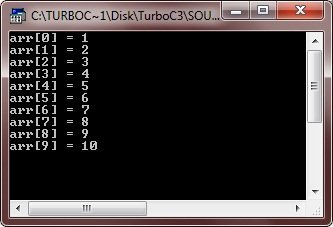Here is one more example program of one-dimensional array:

```/* C Arrays - One-dimensional Array Program */

#include<stdio.h>
#include<conio.h>
void main()
{
int arr;
int i;
clrscr();

printf("Enter 10 array elements: ");
for(i=0; i<10; i++)
{
scanf("%d", &arr[i]);
}

printf("\nThe elements of array arr are: \n");
for(i=0; i<10; i++)
{
printf("%d\n", arr[i]);
}

getch();
}```

Here is the sample run of this C program: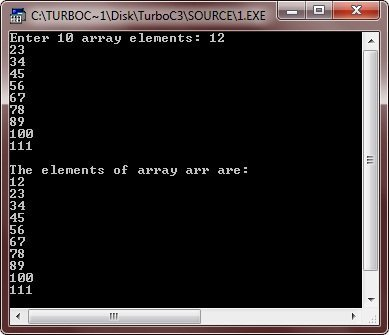## Two-dimensional Arrays in C

C language also supports multi-dimensional arrays. Two-dimensional array is the simplest form of multi-dimensional array. Here is the general form to declare two-dimensional array in C:

`int arr;`

You can called two-dimensional array as an array of one-dimensional array. Therefore, from the above declaration, you can say int arr as an array (size 10) of one-dimensional array (size 5). Here first index responsible for rows and the second index responsible for columns. So the above array have 10 rows and 5 columns. Let's take an example.

```/* C Arrays - Two-dimensional Array Program */

#include<stdio.h>
#include<conio.h>
void main()
{
int arr;
int i, j, val=1;
clrscr();

// initializing array
for(i=0; i<10; i++)
{
for(j=0; j<5; j++)
{
arr[i][j] = val;
val++;
}
}

// displaying array
for(i=0; i<10; i++)
{
for(j=0; j<5; j++)
{
printf("arr[%d][%d]=%d\t", i, j, arr[i][j]);
}
printf("\n");
}

getch();
}```

Here is the sample output of this C program: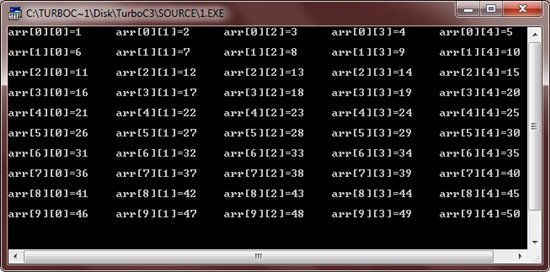Let's look at one more example:

```/* C Arrays - Two-dimensional Array Program */

#include<stdio.h>
#include<conio.h>
void main()
{
int arr;
int i, j;
clrscr();

printf("Enter 5*3 Matrix: ");
for(i=0; i<5; i++)
{
for(j=0; j<3; j++)
{
scanf("%d", &arr[i][j]);
}
}

printf("\nThe Matrix is:\n");
for(i=0; i<5; i++)
{
for(j=0; j<3; j++)
{
printf("%d\t", arr[i][j]);
}
printf("\n");
}

getch();
}```

Below is the sample run of the above C program: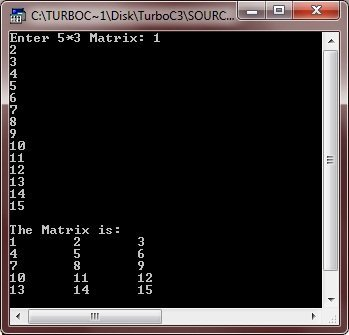## Multi-dimensional Arrays in C

C language allows arrays of more than two dimensions. Here is the general form of a multi-dimensional array declaration:

`type array_name[size1][size2][size3]...[sizeN];`

Arrays of more than three dimensions are not often used because of the amount of memory they require. For instance, a four-dimensional character array with dimensions 10,6,9,4 requires

`10*6*9*4`

or 2,160 bytes. If this array held 2-byte integers, 4,320 bytes would be needed. And if this array held doubles (assuming 8 bytes per double), then 17,280 bytes would be required. The storage required increases exponentially with the number of dimensions. So more than three dimensions array are not often used.

Here is an example illustrating multi-dimensional array in C:

```/* C Arrays - Multi-dimensional Array Program */

#include<stdio.h>
#include<conio.h>
void main()
{
int arr;
int i, j, k, l;
int val=1;
clrscr();

// initializing array
for(i=0; i<1; i++)
{
for(j=0; j<2; j++)
{
for(k=0; k<3; k++)
{
for(l=0; l<4; l++)
{
arr[i][j][k][l] = val;
val++;
}
}
}
}

// displaying array
for(i=0; i<1; i++)
{
for(j=0; j<2; j++)
{
for(k=0; k<3; k++)
{
for(l=0; l<4; l++)
{
printf("arr[%d][%d][%d][%d] = %d\n",i,j,k,l,arr[i][j][k][l]);
}
}
}
}

getch();
}```

Here is the sample output of the above C program: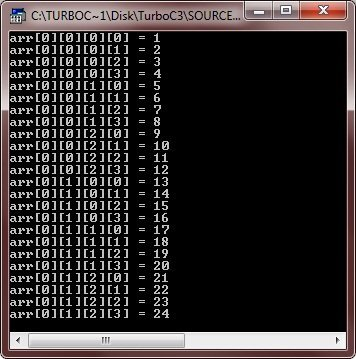## C Arrays Examples

Here are the list of array example programs in C:

Tools
Calculator